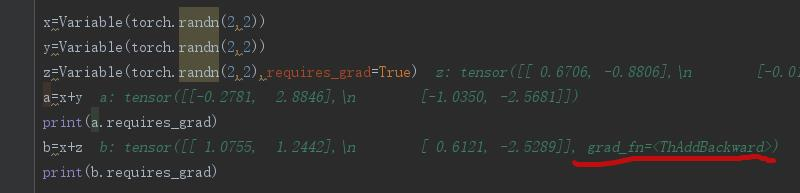# pytorch里optimizer作用、自动求导autograd，from_numpy、numpy()等函数声明变量时候的浅拷贝、显卡内存 out of memory

1、其中optimizer的作用，其主要起到的作用就是进行权重的更新，如下：

net=models.resnet50(pretrained=False)
learning_rate=0.01
# 权重更新 如果使用自带的optimizer函数进行梯度更新，效率更好，因为其还可以引入梯度
#下降的方法，例如动量等方法
for f in net.parameters():

# 使用optimizer进行更新的方法
import torch.optim as optim
inputdata=[1,2,3]
criterion=torch.nn.CrossEntropyLoss()
optimizer=optim.SGD(net.parameters(),lr=0.01)
output=net(inputdata)
loss=criterion(output,inputdata)
# 进行反向梯度计算
loss.backward()
# 进行权重更新
optimizer.step()

    optimizer_ft = optim.SGD(model_ft.parameters(), lr=0.03, momentum=0.9)

A、

# 自动求导
# 自动求导
from torch.autograd import Variable

x = torch.ones(1)
x = Variable(x, requires_grad=True)
y = x * 2

y.backward()


tensor([2.])

x=Variable(torch.randn(2,2))
y=Variable(torch.randn(2,2))
a=x+y
b=x+z
print(b.requires_grad)

False
TrueRuntimeError: element 0 of tensors does not require grad and does not have a grad_fn

out_selected_a = Variable(torch.from_numpy(out_a.cpu().data.numpy()[hard_triplets]).cuda(),requires_grad=True)
triplet_loss = TripletMarginLoss(args.margin).forward(out_selected_a, out_selected_p,  out_selected_n).cuda()
triplet_loss.backward()

B、

model = models.resnet50(pretrained=True)
# 冻结部分的层的权重
for params in model.parameters():
model.fc = torch.nn.Linear(512, 1000)
# 定义优化器,这里虽然传了全部模型的参数，但是其由于上面冻结了一些层，所以这里只会更新最后的全连层
optomizer = torch.optim.SGD(model.parameters(), lr=1e-2, momentum=0.9)

# 另一种冻结模型的方法：传给优化器需要更新的网络参数即可
model=models.resnet50(pretrained=True)
optomizer=torch.optim.SGD(model.fc.parameters(),lr=1e-2,momentum=0.9)

3、关于numpy与torch里的数据类型转换问题：

n1 = np.array([1., 2.], dtype=np.float32)
n2 = np.array([1., 2.], dtype=np.int32)
# 其中from_numpy不会进行数据的深拷贝
t1 = torch.from_numpy(n1)
# 这里的是把float型转换为torch里的float型,由于数据类型相同不会进行深拷贝
t2 = torch.FloatTensor(n1)
#这里把int型转换为torch里的float型，由于数据类型不同会进行深拷贝
t3 = torch.FloatTensor(n2)
print("n1:", n1)
print("n2", n2)
print("t1", t1)
print("t2", t2)
print("t3", t3)
n1 = 5.
print("t1_size:", t1.size())
print("n2:", n2)
print("t1:", t1)
print("t2:", t2)
print("t3", t3)
print("计算完成")

注意：这里的如果数据类型相同则不会进行深拷贝，如果不同则会进行深拷贝。

b、关于tensor转换成numpy，其情况主要分为两类：

#其中data主要是为了再train模式不报错。而在valid模式下要不要data都无所谓，所以统一都加data.
data1=out_a.cpu().data.numpy()

RuntimeError: Can't call numpy() on Variable that requires grad.
Use var.detach().numpy() instead.

 data1=out_a.numpy()

4、模型训练的时候报cuda rumtime error(2): out of memory

total_loss = 0
for i in range(10000):
output = model(input)
loss = criterion(output)
#进行反向传播的时候要用具有历史记录的loss进行传播
loss.backward()
optimizer.step()
#这里记录的total_loss是为了计算平均损失的，所有这里要改为total_loss += float(loss)
total_loss += loss

for i in range(5):
intermdeiate = f(input[i])
result += g(intermediate)
output = h(result)
return output